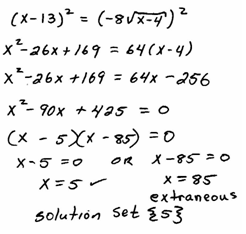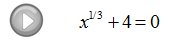## Pages

Now that we have learned how to work with radical expressions, we next move on to solving.

Use caution when solving radical equations because the following steps may lead to extraneous solutions, solutions that do not solve the original equation.

Solve:Step 2: Square both sides of the equation.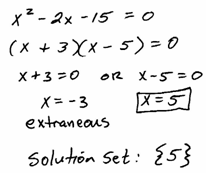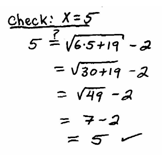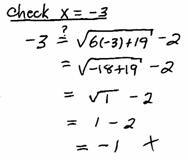Whenever you raise both sides of an equation to an even power, you introduce the possibility of extraneous solutions so the check is essential here.

Solve.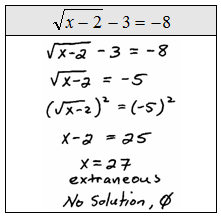The index determines the power to which we raise both sides.  For example, if we have a cube root we will raise both sides to the 3rd power. The property that we are using isfor integers n > 1 and positive real numbers x. After eliminating the radical, we will most likely be left with either a linear or a quadratic equation to solve.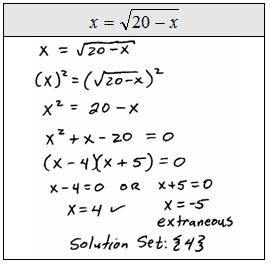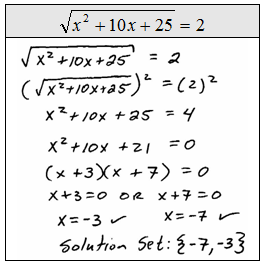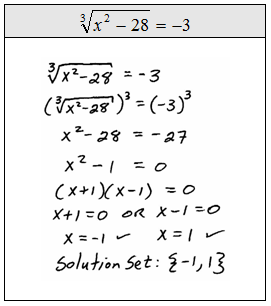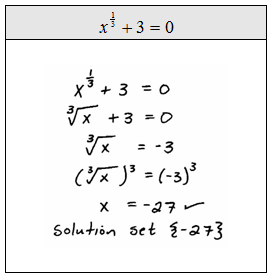The check mark indicates that we have actually checked that the value is a solution to the equation, do not dismiss this step, it is essential.Some radical equations have more than one radical expression.  These require us to isolate each remaining radical expression and raise both sides to the nth power until they are all eliminated.  Be patient with these, go slow and avoid short cuts.
Solve: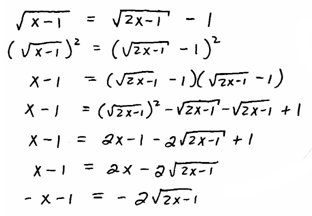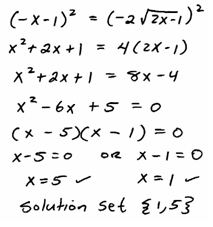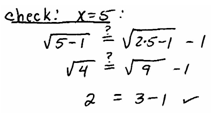Solve: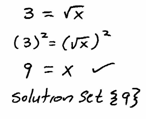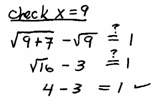Solve: